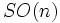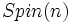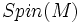# Spin structure

## Definition

A spin structure on an oriented Riemannian manifold is a lift of its gluing cocycle to$SO(n)$, to a gluing cocycle to$Spin(n)$, the double cover of$SO(n)$.

Two spin structures are said to be equivalent if Fill this in later

The set of isomorphism classes of spin structures on a Riemannian manifold$M$ is denoted as$Spin(M)$.